## Example Questions

### Example Question #162 : Fractions

Solve for x where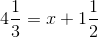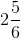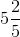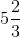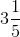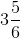Explanation:

To solve this, subtract 1 1/2 from both sides. Convert to common denominators.

4 1/3 – 1 1/2 = 4 2/6 – 1 3/6.

In order to subtract, you'll want to "borrow" from the 4 2/6. Rewrite 4 2/6 as 3 8/6 and then subtract 1 3/6 from this. Your solution is 2 5/6. Most calculators will also do these calculations for you.

### Example Question #163 : Fractions

Solve for: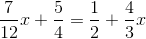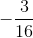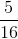Explanation: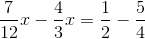Next, you need to find the common denominator. For the left side of your equation, it is. For the right, it is. This means that you need to rewrite as follows: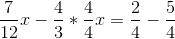Now, simplify and combine terms: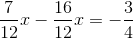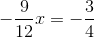You can further simplify the left side: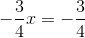Next, multiply both sides by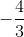. This gives you: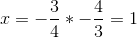### Example Question #164 : Fractions

Simplify: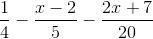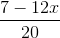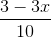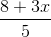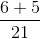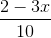Explanation:

First, you must convert your fractions to the common denominator of: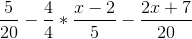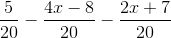Next, you must be very careful. Notice how you must handle your subtractions in order to maintain the correct distribution of signs: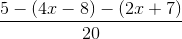Now, carefully distribute for each group: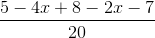Next, simplify: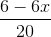Factor out the commonin the numerator: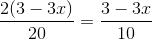There is still a common, but that does not help you get your fraction into the form found in the answers.

### Example Question #165 : Fractions

Choose the answer which best solves the equation below: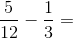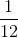Explanation:

To solve this equation, you must first make sure that both fractions have a common denominator.

In this case the common denominator will be 12: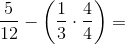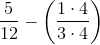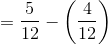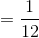### Example Question #166 : Fractions

If John hasslices of anslice pizza left over, and he eats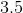of them, what fraction of the pizza does he have left over?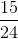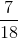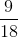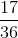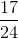Explanation:

To find this answer, first you need to set up your equation: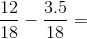And you need to get rid of the decimal in the numerator. We can do this by multiplying each fraction by 2/2.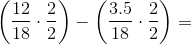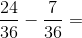### Example Question #1 : How To Subtract Fractions

What common number can you add to the numerator and denominator ofto get?Explanation:

Set up an equation where you add the same unknown number (x) to both the numerator and the denominator of the original fraction, and set the equation equal to.Cross-multiply the fractions to simplify.Now, solve for x.